Mobile QR Code1. (Department of Electronic Engineering, Gachon University, Sujeong-gu, Seongnam-si, Gyeonggi-do 13120, Korea)

One-transistor dynamic random-access memory (1T DRAM), 3-D stack array, capacitor truncation, data retention, carrier diffusion, error function, continuity equation

## I. INTRODUCTION

In order to obtain higher memory density and three-dimensional (3-D) array architecture, great deal of effort has been made to eliminate the capacitor in the conventional dynamic random-access memory (DRAM) in the one-transistor one-capacitor (1T1C) cell structure (1-3). Additionally, by truncating the capacitor, operation speed and processing simplicity can be significantly enhanced (4). On the other hand, it has been known that 1T DRAM has a demerit of rather short retention time and lacks of competitiveness against the conventional DRAM (5). In this work, where the most fundamental reason for the shorter data retention time comes from is analytically studied, out of the major determining factors of carrier diffusion, drift, and recombination. The holes generated by the program operation in 1T DRAM are accurately traced with regard to both position and time. It has been found out that diffusion is the most predominant factor in determining the data retention of 1T DRAM, which can provide a highly practical consideration to be made in performing the optimal design of 1T DRAM devices.

Fig. 1. Device framework for the simulation (a) Schematic of the device structure, (b) Hole distribution after a program operation.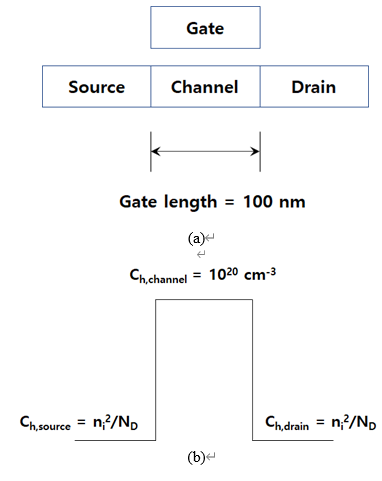## II. SIMULATION APPROACH AND PHYSICS

Fig. 1(a) shows the schematic of the assumed 1T DRAM device simply based on an n-type metal-oxide-semiconductor field-effect transistor (MOSFET) transistor. The doping concentrations of source and drain junctions are both n-type 10$^{20}$ cm$^{-3}$, and that of channel is p-type 10$^{18}$ cm$^{-3}$. It has been assumed the p-type channel is populated with excess holes in the concentration of 10$^{\mathrm{20~}}$cm$^{-3}$ after a program operation. In recent reports, it is noted that program operation can be performed by band-to-band tunneling for higher energy efficiency and device reliability rather than by impact ionization (6). Fig. 1(b) shows the hole concentration over the entire device region, encompassing source, channel, and drain, and it was assumed that concentration of holes as the minority carriers in the source and drain can be simply calculated by the mass action law. c$_{\mathrm{h}}$ is hole concentration, ni is the intrinsic carrier concentration at 300 K = 1.5 ${\times}$ 10$^{10}$ cm$^{-3}$, and N$_{\mathrm{D}}$ is donor concentration = 10$^{20}$ cm$^{-3}$. By the hole concentration values in Fig. 1(b), superposition of the functions describing the diffusion which can take place only by the gradient in carrier concentration allows the numerical calculation of position of carriers at a specific time. The concentration function is the sum of two functions over step concentration profiles as shown in Fig. 2. The summed function describes the programmed holes confined in the channel at the initial moment right after a program operation is performed. The left-hand-side step function is c(x, t = 0) = 0 for -∞ < x < 0 and c(x, t = 0) = c0 for 0 < x < ∞. Also, the right-hand-side one depicts c(x, t = 0) = 0 for -∞ < x < Δx and c(x, t = 0) = -c0 for Δx < x < ∞. The negative concentration value does not have a physical meaning at this stage but it gives the (nearly) zero hole concentration in the drain junction when summed with the left-hand-side one. This sum of two step functions provides the superposition function for time- and position-dependent hole concentration distribution.} A close look into the carrier concentrations in the channel reveals that the hole concentration is very high right after a program operation is performed and there are only a few mobile electrons in the channel. Once the thermal equilibrium is broken after a program operation and high injection of holes into the channel is established, the hole concentration becomes extremely high compared with the concentration of electron as the minority in the p-type channel. The recombination rate is negatively proportional to sum of the mobile electrons and holes, as the sum of steady-state and transient excess carriers, the recombination rate is very high right after a program operation. However, considering that the programmed holes are accumulated in the p-type channel, the number of electrons as the recombination center for the mobile holes is extremely small as can be simply predicted by mass action law (even with a correction in case of high carrier concentration). Thus, it is hardly expected that the recombination of programmed holes in the p-type channel does not make a significant reduction in the number of holes, i.e., majority and programmed carriers at all. The threshold voltage elasticity, or equivalently, high-to-low read current restoring is determined not by the recombination speed but the amount of recombination itself. Therefore, when it comes to the case that p-type channel is utilized for the hole storage, the recombination cannot be a competing factor. For these reasons, the number of holes vanishing by recombination is extremely small compared with the total number of holes (holes as the majority carrier and the excess holes), and consequently, recombination cannot be considered in the following simulations (7). Fig. 3(a) through (d) shows the energy-band diagrams under change with time, being subordinate to the redistribution of holes by diffusion. Fig. 3(a) presents the steady-state energy-band diagram before a program operation. The holes from the doped acceptors are located in the channel and the Fermi levels across source, channel, and drain are aligned showing invariance with position. There is no net hole conduction by the dynamic equilibrium between hole diffusion and drift. After a program operation is performed, a number of holes are injected into the channel. It can be assumed that the program operation can be achieved by band-to-band tunneling for higher energy efficiency and device reliability compared with the case by impact ionization (6). The valence electrons are tunneled into the drain leaving the mobile holes behind in the 1T DRAM channel. Thus, once the program operation is carried out, the thermal equilibrium is instantly broken by the high-level hole injection and the center of the bands are dragged down by the positive potential effectively applied by the high concentration of holes as schematically shown in Fig. 3(b). Although the center of the bands might be higher than those of the source and drain junctions depending on how many holes are injected per program operation, it should be an unchanged fact that the higher concentration of holes has higher probability of overcoming the lowered potential energy barriers and escaping out of the channel. As time passes, the potential energy barriers seen by the channel holes toward the source and drain junctions become deeper as shown in Fig. 3(c) and (d). The electric fields constructed at source/channel and channel/drain metallurgical junctions act against the hole diffusion but are weakened as time passes. No matter how high or low the electric field might be, the field hinders the stored holes from diffusing out of the channel, and finally, a new balance between diffusion and drift is reached (Fig. 3(a)). Until that steady state, the majority of driving force in hole redistribution should be diffusion mechanism, which can be clarified by the analytical equation solving in the following chapters.

Fig. 2. Hole concentration profile described by a superposition of two step functions on both sides of the p-type channel.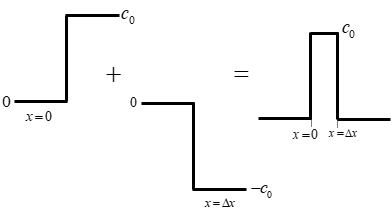Fig. 3. Time-dependent alteration in energy-band diagram after a program operation. Energy-band diagrams (a) thermal equilibrium before a program operation, (b) right after a program operation, (c) after a short time passes, (d) after a certain amount of time is further elapsed (but before steady state).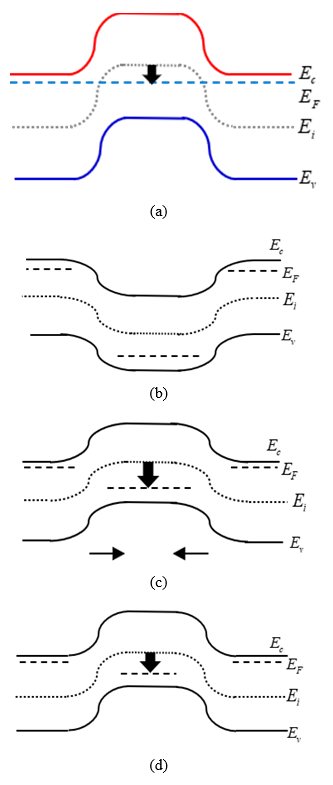Fig. 4. Distribution of hole concentration (a) as a function of position across the entire 1T DRAM junctions at different times, (b) as a function of time at the channel center and the edge.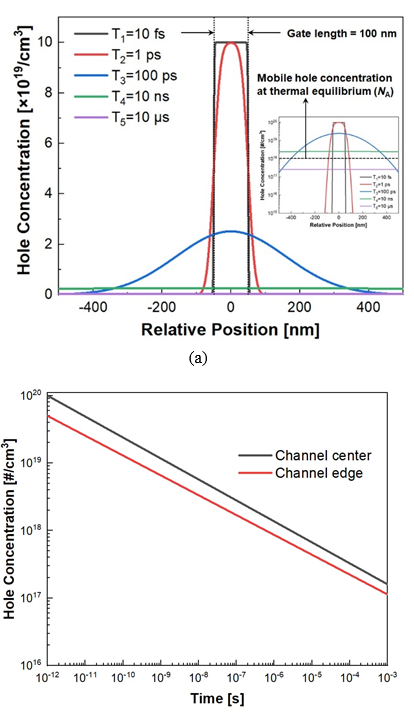## III. MATHEMATICAL FOUNDATION AND ANALYTICAL SIMULATION RESULTS

Eq. (1) can mathematically describe the hole concentration in the 1T DRAM channel and can be solved by a proper set of boundary conditions that can be derived from the values given in Fig. 2(a) and (b) (8).

##### (1)
$$c_{h}(x, t)=\frac{c_{i b}}{2}\left[\operatorname{erf}\left(\frac{x+\Delta x / 2}{\sqrt{4 D t}}\right)-\operatorname{erf}\left(\frac{x-\Delta x / 2}{\sqrt{4 D t}}\right)\right]$$

Here, $\textit{c}$$_{h}(\textit{x},\textit{t}) is the position- and time-dependent hole concentration and \textit{c}$$_{h0}$ denotes the peak hole concentration at the channel center right after a program operation. \textit{erf} is error function, $\textit{x}$ is the position coordinating $\textit{x}$ = 0 at the channel center, and $\textit{D}$ is the diffusion coefficient of hole. ${\Delta}$$\textit{x} is the channel length so that {\Delta}$$\textit{x}$/2 = 50 nm. Fig. 4(a) depicts the distribution of hole concentration across the 1T DRAM device as a function of position at different moments of time, from 10 fs to 10 ${μ}$s. Being reminded of the fact that the thermal equilibrium is reached when the hole concentration at the channel center is equal to the acceptor doping concentration N$_{\mathrm{A}}$ = 10$^{18}$ cm$^{-3}$, the equilibrium can be achieved in an extremely short time below 10 ${μ}$s as can be inferred by Fig. 4(a). The recombination time is ranged from nanosecond to a few milliseconds depending on channel material status, and for the clean Si substrate, it might reach a few milliseconds. The recombination is a spontaneous process that takes place in semiconductors and cannot be controlled unless a precise electrical manipulation is schemed. In other words, additional power consumption can be required for longer traveling time without recombination. Fig. 4(b) depicts the hole concentration as a function of time at different positions, at the channel center and at the edge, by which it is confirmed that diffusion is dominating over recombination since the time to thermal equilibrium by diffusion is substantially shorter than a few milliseconds in clean Si, that by recombination. Removal of the term for recombination current and dividing both sides by q gets the full current continuity equation back to the Fick’s law as shown in Eq. (2)(9).

##### (2)
$$\frac{\partial c}{\partial t}=-\frac{1}{q} \frac{\partial J}{\partial x}$$

##### (3)
$$\frac{\partial}{\partial x}\left[\mu_{p} c E(x, t)\right]=-\frac{\partial c}{\partial t}+D \frac{\partial^{2} c}{\partial x^{2}}$$

Eq. (2) implies the flux of particle in the unit of particles per unit volume per unit time. $\textit{c}$ is the volume density of particles and it can be interpreted as $\textit{c}$$_{h}$($\textit{x}$,$\textit{t}$) in Eq. (1) in this problem. If diffusion and drift terms are substituted into the hole current density $\textit{J}$ in Eq. (2), the term for hole flux by drift can be extracted by Eq. (3). Once the solution to Eq. (1) is obtained and plugged into Eqs. (2) and (3) the hole flux by drift can be simply obtained without the complicated procedure of obtaining the internal electric fields. Fig. 5 demonstrates total hole flux, hole flux by diffusion, hole flux by drift at the same time as a function of time. It is explicitly revealed that the hole flux by dfift is negligibly small compared with that by diffusion, which confirms that the total hole flux is not distinguishable from hole flux by diffusion in Fig. 5(10). There are two competing factors in determining the threshold voltage when additional holes are injected into the p-type channel: (1) source-to-channel intrinsic barrier height elevation and (2) body potential elevation leading to a channel-to-source forward bias. Between these two factors, the second one has the predominance over the first one, which can be found in the partially-depleted (PD) silicon-on-insulator (SOI) MOSFET characteristics. In the PD SOI n-type MOSFET, the holes generated by impact ionization has the effect of elevating the body potential and lowering the threshold voltage although higher hole concentration in the p-type region will heighten the intrinsic potential barrier. Although the mechanism in this work has been altered from impact ionization to band-to-band tunneling, the results can be presumably understood to be the same. Although there is no explicit way of quantitatively identifying the relation between the number of programmed holes and threshold shift, a threshold voltage shift within 0.3 V can be predicted form the existing simulation and empirical results at a p-type channel doping concentration of 10$^{18}$ cm$^{-3}$ (11-13). Out of the major mechanisms of diffusion, drift, and recombination, it is proven that diffusion has the predominance among them. Researches have been conducted to increase the retention time of 1T DRAM by at least locally introducing insulating layers between channel (charge storage) and other junctions. Although they were not analytically proven as in this work, the effects of preserving the programmed charges for a longer time could be obtained by introducing the physical barriers (14). Another study was on the effect of preventing the carriers from escaping out of the floating body when an electrical barrier was constructed by a heterostructure between Si and GaP. These physical or electrical barriers substantially increase the retention time in 1T DRAM operation by blocking the diffusion of programmed charges. If the recombination had been the predominant factor, there should have been little effect of elongating the data retention by simply introducing the barriers (16).

Fig. 5. Hole flux as a function of time with discrimination between diffusion and drift components.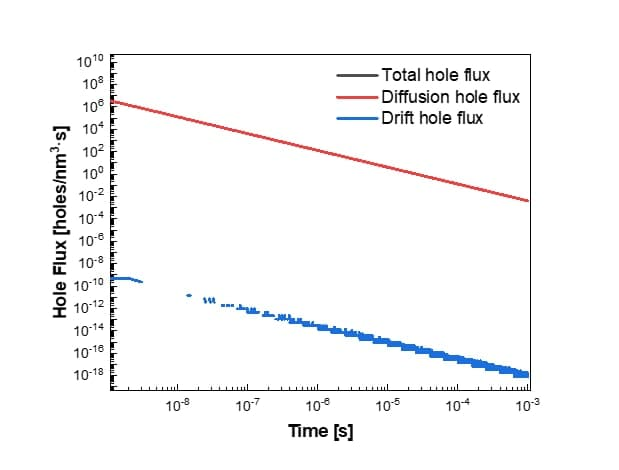## IV. CONCLUSION

Through the mathematical and analytical methods, it has been found that the data retention capability of 1T DRAM device is predominantly determined by diffusion of programmed holes while the effects of recombination and drift are insignificant. In performing the optimal design of various 1T DRAM devices in novel structure, this conclusion needs to be considered for stronger data retention capability in advanced DRAM technologies.

### ACKNOWLEDGMENTS

This work was supported by the Korean Ministry of Trade, Industry and Energy (MOTIE) under Grant 10080513.

### REFERENCES

1
Nguyen Q. T., Jang D., Ansari M. H. R., Kim G., Cho S., Cho I. H., Oct 2021., Reliability improvement of 1T DRAM based on feedback transistor by using local partial insulators, Jpn. J. Appl. Phys.,104002-1-104002-7, Vol. 60, No. 102
Cho S., Ansari M. H. R., Apr 2020, Performance Improvement of 1T DRAM by Raised Source and Drain Engineering, IEEE Trans, Electron Devices, Vol. 67, No. 4, pp. 1471-14793
Navlakha N., Lin J.-T., Kranti A., Apr 2021, Improved Retention Time in Twin Gate 1T DRAM With Tunneling Based Read Mechanism, IEEE Electron Devices, Vol. 68, No. 4, pp. 1577-15844
Yoshida E., Tanaka T., Apr 2006, A Capacitorless 1T-DRAM Technology Using Gate-Induced Drain-Leakage (GIDL) Current for Low-Power and High-Speed Embedded Memory, IEEE Trans. Electron Devices, Vol. 53, No. 4, pp. 692-6975
Lee Y. J., Cho S., 2021, Assessment of Data Retainability in Capacitorless Dynamic Random-Access Memory by Time- and Position-Dependent Hole Diffusion Function, Proc. 2021 Asia-Pacific Workshop on Fundamentals and Applications of Advanced Semiconductor Devices (AWAD 2021)6
Yu E., Cho S., Shin H., Park; B.-G., Apr. 2019, A Band-Engineered One-Transistor DRAM with Improved Data Retention and Power Efficiency, IEEE Electron Device Lett, Vol. 40, No. 4, pp. 562-5657
Schenk. A., May 1992, A model for the field and temperature dependence of Shockley-Read-Hall lifetimes in silicon, Solid-State Electron, Vol. 35, No. 11, pp. 1585-1592.8
Balluffi R. W., Allen S. M., Carter W. C., 2005, Kinetics of Materials, John Wiley & Sons, NJ, USA, pp. 83-869
Burgler J. F., Coughran William M., Jr , Fichtner; W., Oct 1991, An Adaptive Grid Refinement Strategy for the Drift-Diffusion Equations, IEEE Trans. Comput. Aided Des. Integr. Circuits Syst., Vol. 10, No. 10, pp. 1251-125810
MATLAB R2021a , MathWorks, online reference at https://kr.mathworks.com/products/new_products/ latest_features.html11
Lim H., Fossum J. G., Oct. 1983., Threshold Voltage of Thin-Film Silicon-on-Insulator (SOI) MOSFET’s, , Vol. ED-30, No. no. 10, pp. 1244-125112
Wei A., Sherony M. J., Antoniadis; D. A., Feb 1988, Effect of Floating-Body Charge on SOI MOSFET Design, IEEE Trans. Electron Devices, Vol. 45, No. 2, pp. 430-43813
Chan M., Su P., Wan H., Lin C.-H., Fung S. K.-H., Niknejad A. M., Hu C., Ko; and P. K., Jun 2004, Modeling the floating-body effets of fully depleted, partially depleted, and body-grounded SOI MOSFETs, Solid-State Electron., Vol. 48, No. 6, pp. 969-97814
Ha J., Lee J. Y., Kim M., Cho S., Cho; I. H., Apr 2019, Investigation and Optimization of Double-Gate MPI 1T DRAM with Gate-Induced Drain Leakage Operation, J. Semicond. Technol. Sci., Vol. 19, No. 2, pp. 165-17115
Ansari M. H. R., Navlakha N., Lee J. Y., Cho S., Apr 2020, Double-Gate Junctionless 1T DRAM With Physical Barriers for Retention Improvement, IEEE Trans. Electron Devices, Vol. 67, No. 4, pp. 1471-147916
Kim M., Ha J., Han J.-H., Cho S., Cho I. H., Nov. 2018, A Novel One-Transistor Dynamic Random-Access Memory (1T DRAM) Featuring Partially Inserted Wide-Bandgap Double Barrier for High-Temperature Applications, Micromachines, Vol. 9, No. 11, pp. 581-589## Author

Yi Ju Lee is pursuing the B.S. degree in electronic engineering as a Research Undergraduate Student in the Department of Electronic Engineering, Gachon University.

Her research interests include advanced semiconductor memory and logic devices and fabrication process integration.

She is a Student Member of the Institute of Electronics and Information Engineeres of Korea (IEIE).

Seongjae Cho received the B.S. and the Ph.D. degrees in electrical engineering from Seoul National University, Seoul, Republic of Korea, in 2004 and 2010, respectively.

He worked as an Exchange Researcher at the National Institute of Advanced Industrial Science and Technology (AIST), Tsukuba, Japan, in 2009.

Also, he worked as a Postdoctoral Researcher at Seoul National University in 2010 and at Stanford University, CA, USA, from 2010 to 2013.

He joined the Department of Electronic Engineering, Gachon University, Seongnam, Republic of Korea, in 2013, where he is currently working as an Associate Professor.

His current research interests include emerging memory technologies, advanced nanoscale CMOS devices, group-IV photonic devices, memory cells for neuromorphic and memory-centric processor technologies.

He is a Lifetime Member of IEIE and a Senior Member of IEEE.